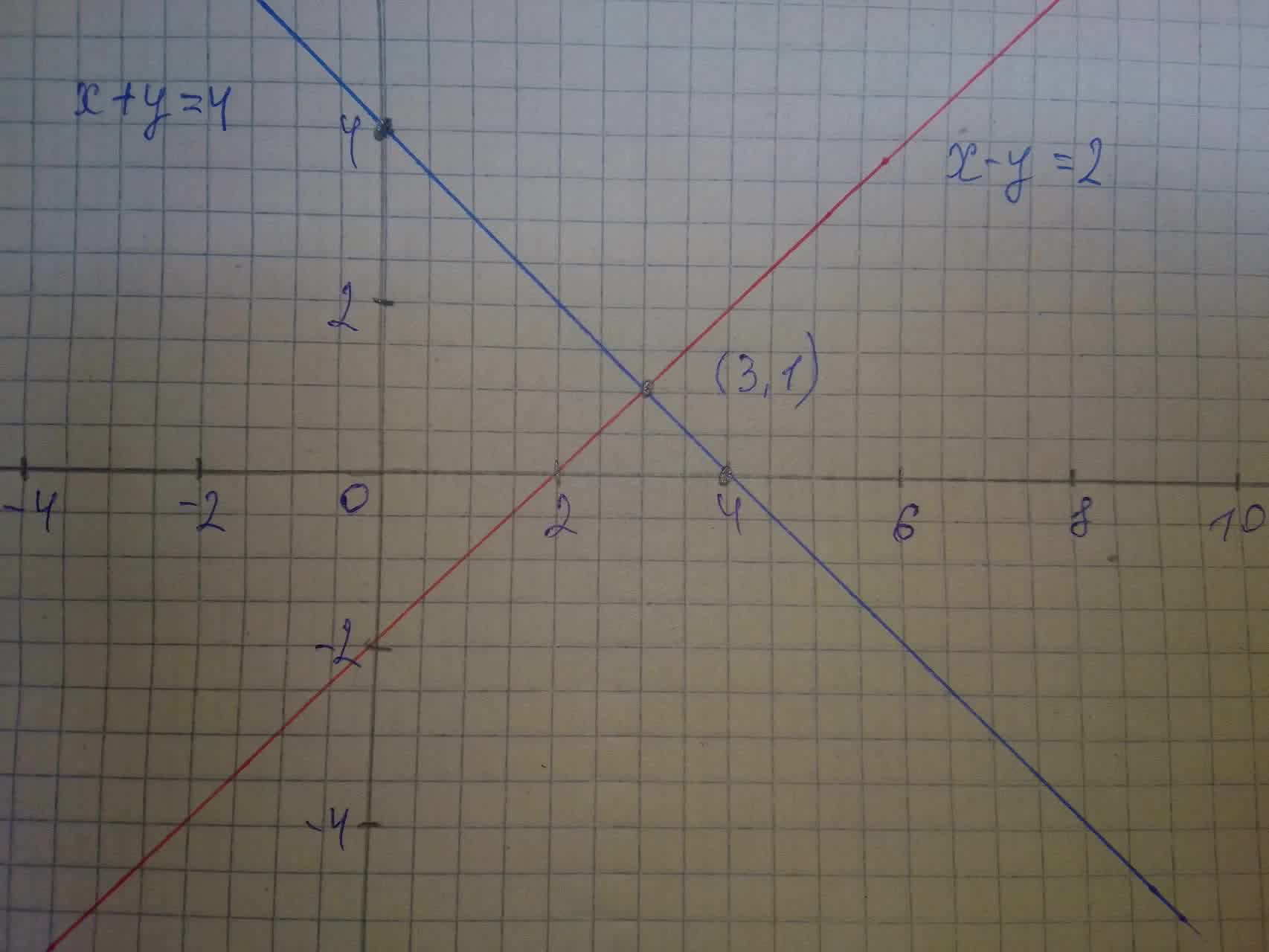Solving Systems Graphically Find or create an example of a system of equations with one solution. Graph and label the lines on a coordinate plane. Provide their equations. State the accurate solution to the system.postillan4 2021-08-04 Answered
Solving Systems Graphically- One Solution
Find or create an example of a system of equations with one solution.
Graph and label the lines on a coordinate plane. Provide their equations.
State the accurate solution to the system.

• Questions are typically answered in as fast as 30 minutes

Solve your problem for the price of one coffee

• Math expert for every subject
• Pay only if we can solve itlobeflepnoumni
Step 1
Consider a system of equation
$$\displaystyle{x}−{y}={2}$$
$$\displaystyle{x}+{y}={4}$$
Step 2The solution of the system is
$$\displaystyle{x}={3}$$
$$\displaystyle{y}={1}$$
Step 3
We can also find the solution of system algebraically
$$\displaystyle{x}−{y}={2}\ldots\ldots.{\left({1}\right)}$$
$$\displaystyle{x}+{y}={4}\ldots\ldots.{\left({2}\right)}$$
From equation (1)
$$\displaystyle{x}−{y}={2}$$
$$\displaystyle{y}={x}−{2}$$
Put the value of y in equation (2)
$$\displaystyle{x}+{y}={4}$$
$$\displaystyle{x}+{x}−{2}={4}$$
$$\displaystyle{2}{x}−{2}={4}$$
$$\displaystyle{2}{x}={4}+{2}$$
$$\displaystyle{2}{x}={6}$$
$$\displaystyle{x}={3}$$
Put the value of x in equation (1)
$$\displaystyle{x}−{y}={2}$$
$$\displaystyle{3}−{y}={2}$$
$$\displaystyle{y}={3}−{2}$$
$$\displaystyle{y}={1}$$
Hence the solution is
$$\displaystyle{x}={3}$$
$$\displaystyle{y}={1}$$